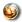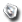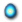Generic template housing different icons. Enter `{{Icon|<variable>}}` to call the template. Here are the icons you can get:

orb =stone =point =common, C, c =uncommon, UC, uc =rare, R, r =superrare, SR, sr =wood =fire =earth =metal =water =light =dark =am =as =eu =zone =battle =hunt =event =yes =no =question =firstStrike =armorUp =armorBk =counter =crit =stop =strength =strHP =strDn =speedUp =speedHP =speedEle =Pencil =If an invalid parameter is passed, you'll get